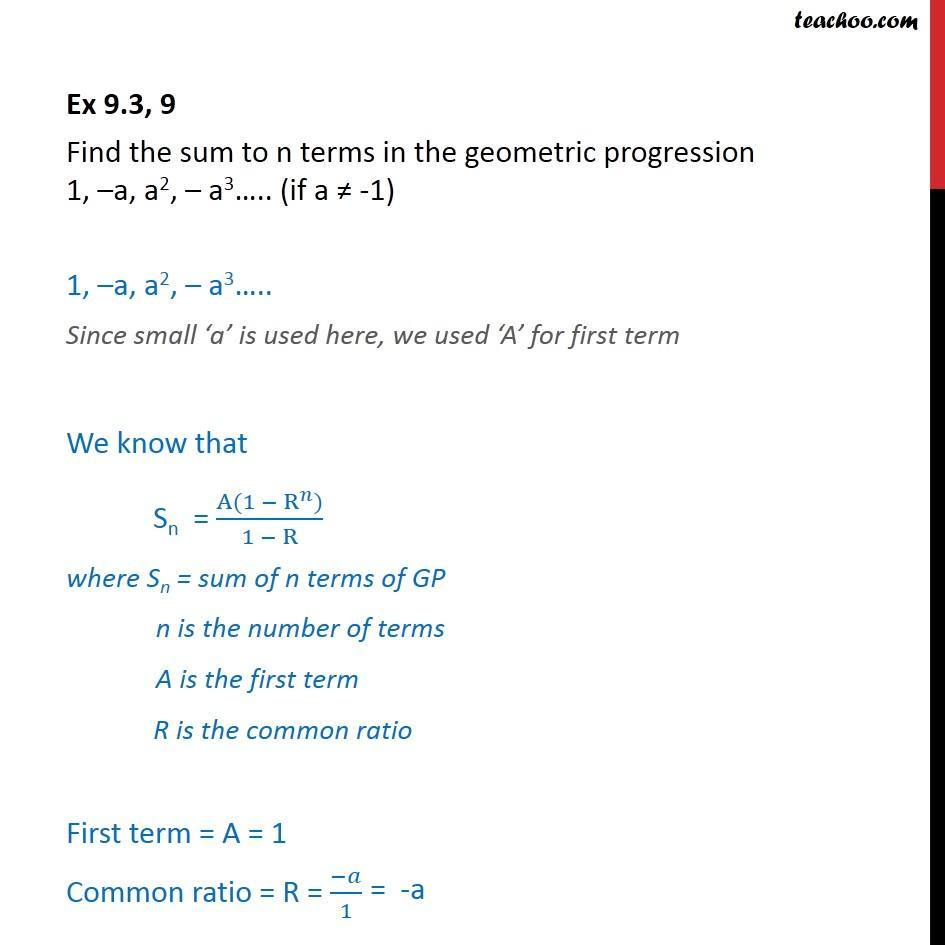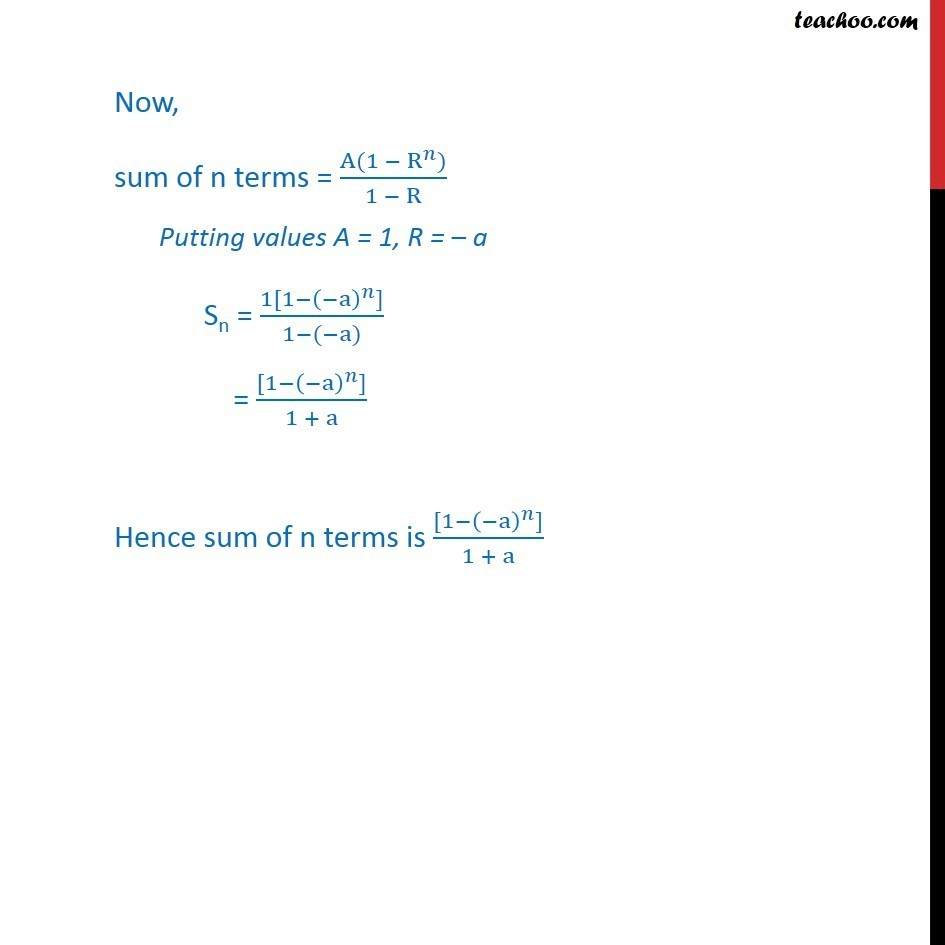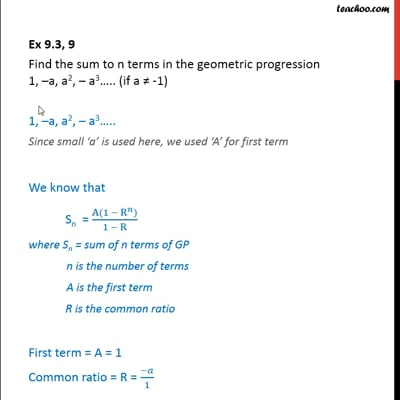Ex 9.3

Chapter 9 Class 11 Sequences and Series
Serial order wiseThis video is only available for Teachoo black users

Maths Crash Course - Live lectures + all videos + Real time Doubt solving!

### Transcript

Ex 9.3, 9 Find the sum to n terms in the geometric progression 1, –a, a2, – a3….. (if a ≠ -1) 1, –a, a2, – a3….. Since small ‘a’ is used here, we used ‘A’ for first term We know that Sn = (A(1 − R^𝑛))/(1 − R) where Sn = sum of n terms of GP n is the number of terms A is the first term R is the common ratio First term = A = 1 Common ratio = R = (−𝑎)/1 Now, sum of n terms = (A(1 − R^𝑛))/(1 − R) Putting values A = 1, R = – a Sn = (1[1−(−a)^𝑛])/(1−(−a)) = ([1−(−a)^𝑛])/(1 + a) Hence sum of n terms is ([1−(−a)^𝑛])/(1 + a)